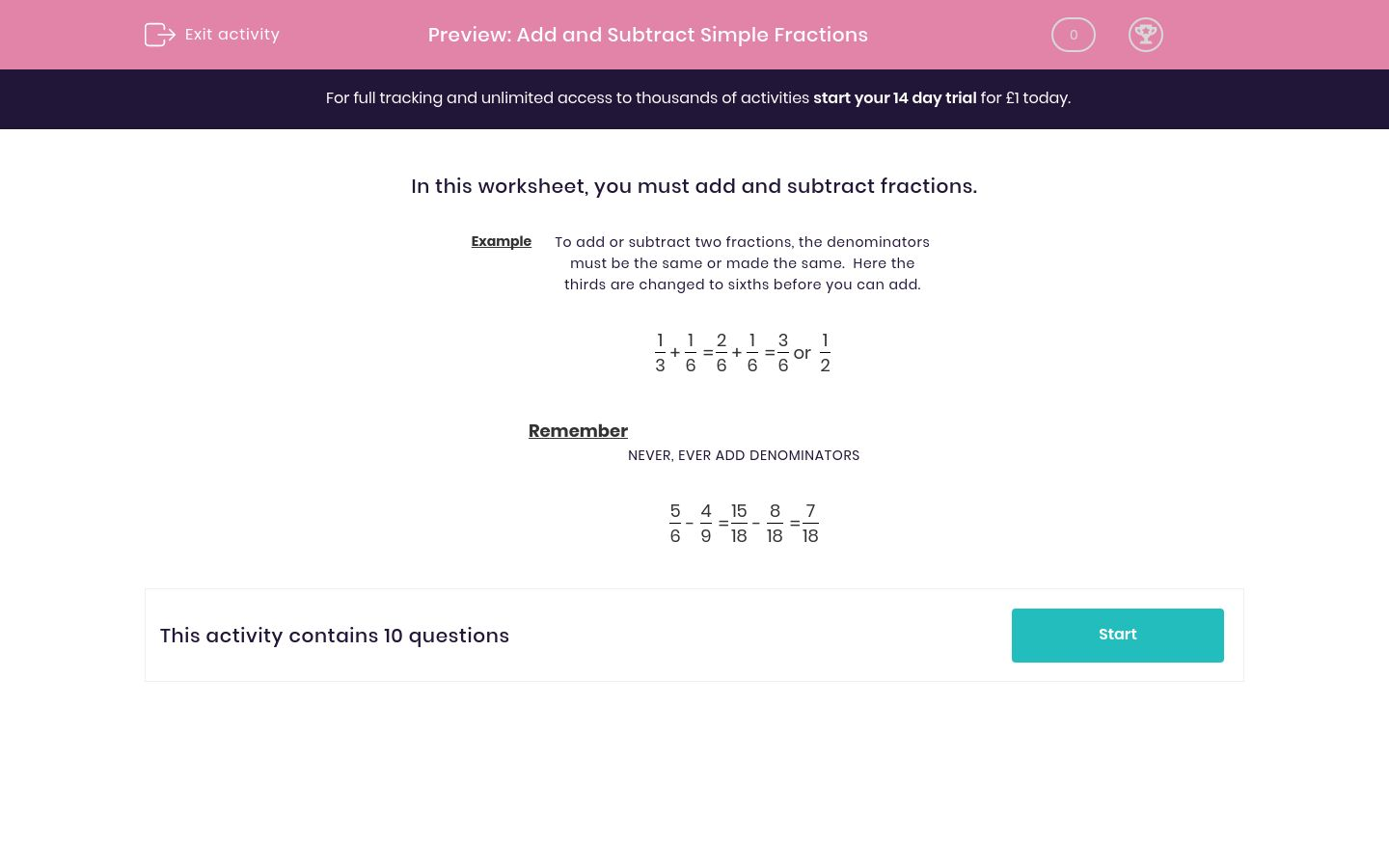# Add and Subtract Simple Fractions

In this worksheet, students improve their skills at adding and subtracting simple fractions.Key stage:  KS 2

Curriculum topic:   Maths and Numerical Reasoning

Curriculum subtopic:   Fractions

Difficulty level:### QUESTION 1 of 10

In this worksheet, you must add and subtract fractions.

Example

To add or subtract two fractions, the denominators must be the same or made the same.  Here the thirds are changed to sixths before you can add.

 1 3
+
 1 6
=
 2 6
+
 1 6
=
 3 6
or
 1 2

Remember

 5 6
-
 4 9
=
 15 18
-
 8 18
=
 7 18

To find the common denominator, find the lowest common multiple of the denominators.

 4 8
+
 1 12
=
 A
 B
+
 C
 D
=
 E
 F
 A B C D E F 26 2 24 12 14

To find the common denominator, find the lowest common multiple of the denominators.

 3 8
+
 1 6
=
 A
 B
+
 C
 D
=
 E
 F
 A B C D E F 26 4 24 13 9

To find the common denominator, find the lowest common multiple of the denominators.

 1 10
+
 1 7
=
 A
 B
+
 C
 D
=
 E
 F
 A B C D E F 7 70 10 50 17

To find the common denominator, find the lowest common multiple of the denominators.

 3 11
 1 6
=
 A
 B
 C
 D
=
 E
 F
 A B C D E F 18 77 11 66 7

To find the common denominator, find the lowest common multiple of the denominators.

 2 10
+
 1 6
=
 A
 B
+
 C
 D
=
 E
 F
 A B C D E F 5 30 40 6 11

To find the common denominator, find the lowest common multiple of the denominators.

 3 7
+
 5 12
=
 A
 B
+
 C
 D
=
 E
 F
 A B C D E F 71 85 84 36 35

To find the common denominator, find the lowest common multiple of the denominators.

 5 8
+
 5 7
=
 A
 B
+
 C
 D
=
 E
 F
 A B C D E F 40 75 35 56 66

To find the common denominator, find the lowest common multiple of the denominators.

 5 6
+
 5 10
=
 A
 B
+
 C
 D
=
 E
 F
 A B C D E F 40 15 30 25 36

To find the common denominator, find the lowest common multiple of the denominators.

 4 12
 3 11
=
 A
 B
 C
 D
=
 E
 F
 A B C D E F 8 44 131 36 132

To find the common denominator, find the lowest common multiple of the denominators.

 1 12
+
 3 11
=
 A
 B
+
 C
 D
=
 E
 F
 A B C D E F 47 11 131 36 132
• Question 1

To find the common denominator, find the lowest common multiple of the denominators.

 4 8
+
 1 12
=
 A
 B
+
 C
 D
=
 E
 F
 A B C D E F 26 2 24 12 14
• Question 2

To find the common denominator, find the lowest common multiple of the denominators.

 3 8
+
 1 6
=
 A
 B
+
 C
 D
=
 E
 F
 A B C D E F 26 4 24 13 9
• Question 3

To find the common denominator, find the lowest common multiple of the denominators.

 1 10
+
 1 7
=
 A
 B
+
 C
 D
=
 E
 F
 A B C D E F 7 70 10 50 17
• Question 4

To find the common denominator, find the lowest common multiple of the denominators.

 3 11
 1 6
=
 A
 B
 C
 D
=
 E
 F
 A B C D E F 18 77 11 66 7
• Question 5

To find the common denominator, find the lowest common multiple of the denominators.

 2 10
+
 1 6
=
 A
 B
+
 C
 D
=
 E
 F
 A B C D E F 5 30 40 6 11
• Question 6

To find the common denominator, find the lowest common multiple of the denominators.

 3 7
+
 5 12
=
 A
 B
+
 C
 D
=
 E
 F
 A B C D E F 71 85 84 36 35
• Question 7

To find the common denominator, find the lowest common multiple of the denominators.

 5 8
+
 5 7
=
 A
 B
+
 C
 D
=
 E
 F
 A B C D E F 40 75 35 56 66
• Question 8

To find the common denominator, find the lowest common multiple of the denominators.

 5 6
+
 5 10
=
 A
 B
+
 C
 D
=
 E
 F
 A B C D E F 40 15 30 25 36
• Question 9

To find the common denominator, find the lowest common multiple of the denominators.

 4 12
 3 11
=
 A
 B
 C
 D
=
 E
 F
 A B C D E F 8 44 131 36 132
• Question 10

To find the common denominator, find the lowest common multiple of the denominators.

 1 12
+
 3 11
=
 A
 B
+
 C
 D
=
 E
 F
 A B C D E F 47 11 131 36 132
---- OR ----

Sign up for a £1 trial so you can track and measure your child's progress on this activity.

### What is EdPlace?

We're your National Curriculum aligned online education content provider helping each child succeed in English, maths and science from year 1 to GCSE. With an EdPlace account you’ll be able to track and measure progress, helping each child achieve their best. We build confidence and attainment by personalising each child’s learning at a level that suits them.

Get started